Technical Article

# LTspice Lab: Buck Converter Current and Voltage Dynamics

August 09, 2023 by Robert Keim

## Improve your understanding of switch-mode voltage regulators by examining the electrical characteristics of a step-down converter during the on and off portions of its switching cycle.

In the preceding article, Understanding Switch-Mode Regulation: The Buck Converter, I introduced and explained the LTspice implementation of the power stage of a step-down switching regulator illustrated in Figure 1.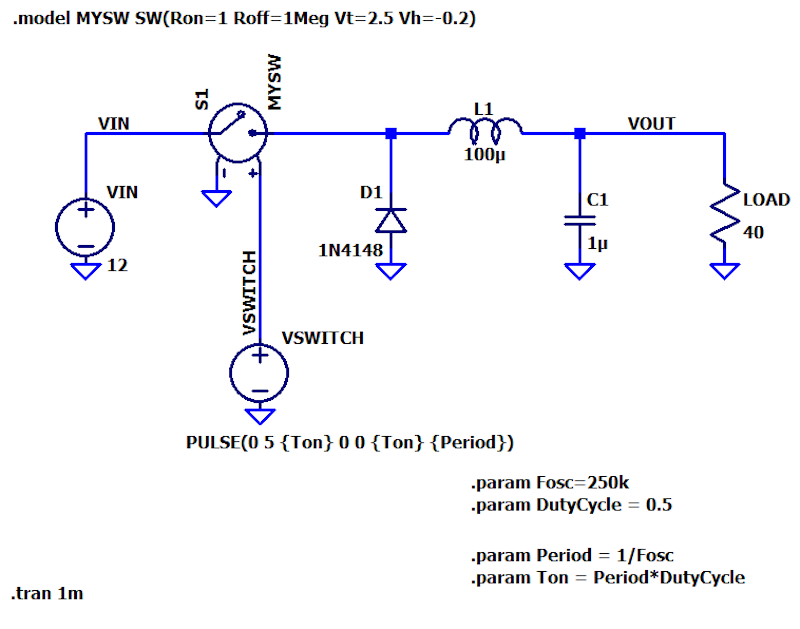##### Figure 1. Buck (step-down) converter simulation schematic

This article will investigate the circuit’s electrical activity relative to the two states of the switch—On and Off.

### Buck Converter Current and Voltage in the On State

When the switch-control waveform (VSWITCH in the schematic) is logic high, the switch is turned on. This allows current to flow freely from the input supply to the right-hand portion of the circuit (Figure 2).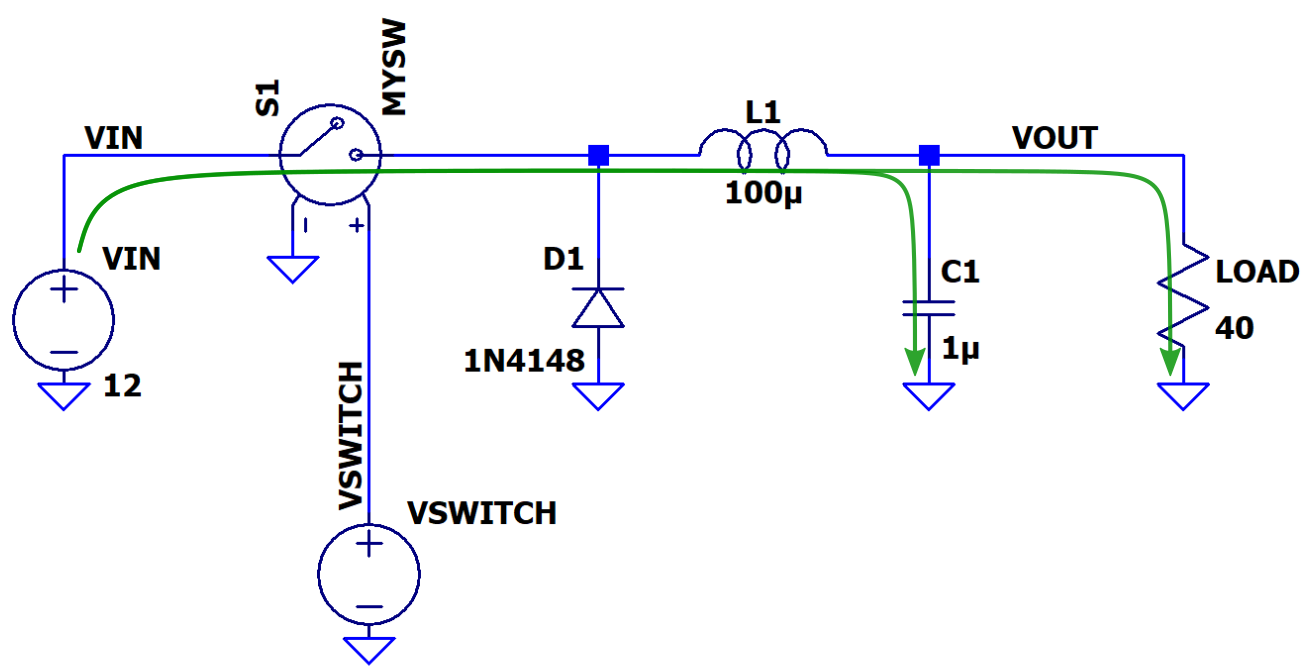##### Figure 2. Buck converter current flow in the On state

As you see in Figure 2, the supply current flows through the switch, S1, and the inductor, L1, on its way to the capacitor, C1, and the load resistor. The current through the inductor is ramping up, and the inductor is “charging,” i.e., the amount of energy stored in its magnetic field is increasing. The inductor current is distributed to the capacitor and to the load.

Note that the diagram does not show any current flowing into the diode. During the On state, the voltage drop across the switch is almost zero, and consequently, the voltage across the diode is approximately equal to VIN. Thus, the diode is reverse-biased and acts like an open circuit.

### Buck Converter Transient Response During Turn On

Let’s carefully consider the information contained in the multi-pane plot of Figure 3 as the switch is turned on. We will discuss each sub-plot beginning at the bottom and working our way to the top.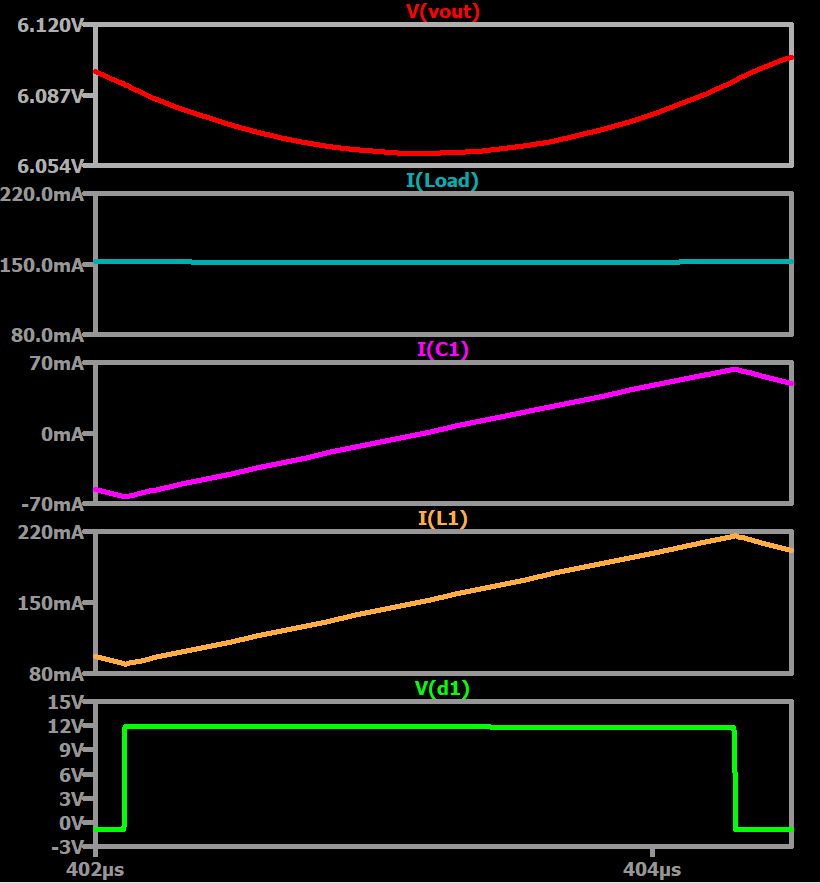#### Diode Voltage

Starting at the bottom, we know we’re in the on state because the voltage (12 V) across the diode, V(d1), equals the input voltage.

#### Inductor Current

The inductor current, I(L1), is ramping up, and the inductor is charging. Note that the minimum value of the vertical axis in this plot is 80 mA, not 0 mA. Though the switch completely blocks input current in the off state, the inductor ensures that significant amounts of current continue to flow in the right-hand portion of the circuit.

#### Capacitor Current

The current flowing into the capacitor, I(C1), is also increasing. The capacitor begins to charge (and its voltage increases) when I(C1) crosses 0 mA and becomes positive.

The load current, I(Load), is stable at the average value of the inductor current. How does the load current remain so stable when the inductor current ramps up and down with 140 mA of ripple? The only two pathways for the inductor current are the load resistance and capacitor C1, so the answer must involve C1.

If you ponder the I(C1) plot, you will see that the capacitor continuously compensates for the inductor current’s deviations. For example, when I(L1) is 80 mA, I(C1) is –70 mA, where the negative sign means that the capacitor is supplying 70 mA. The load gets 80 mA from the inductor plus 70 mA from the capacitor, resulting in total current of 150 mA.

However, when I(L1) is 220 mA, I(C1) is +70 mA, where the positive sign means that the capacitor is absorbing 70 mA. Thus, the load gets 220 mA – 70 mA = 150 mA.

#### Output Voltage

The output voltage, V(vout), which is also the voltage across the capacitor, exhibits a low amplitude ripple around its average voltage. In the plot of the output voltage, I have magnified the y-axis to highlight the voltage ripple.

Note that the voltage begins to increase when the capacitor current crosses 0 mA. This makes sense—in this simulation, positive capacitor current is current that flows into the capacitor and, therefore, causes its voltage to increase.

The approximately 6 V at the output is roughly half of the 12 V input. The buck converter has indeed stepped the voltage down, as desired.

### Buck Converter Current and Voltage in the Off State

When the switch, S1, is off, current continues to flow in the right-hand portion of the circuit, as illustrated in Figure 4. This current can’t come from the input supply, though, and it also can’t come from nowhere. Instead, it circulates, with help from the diode, D1.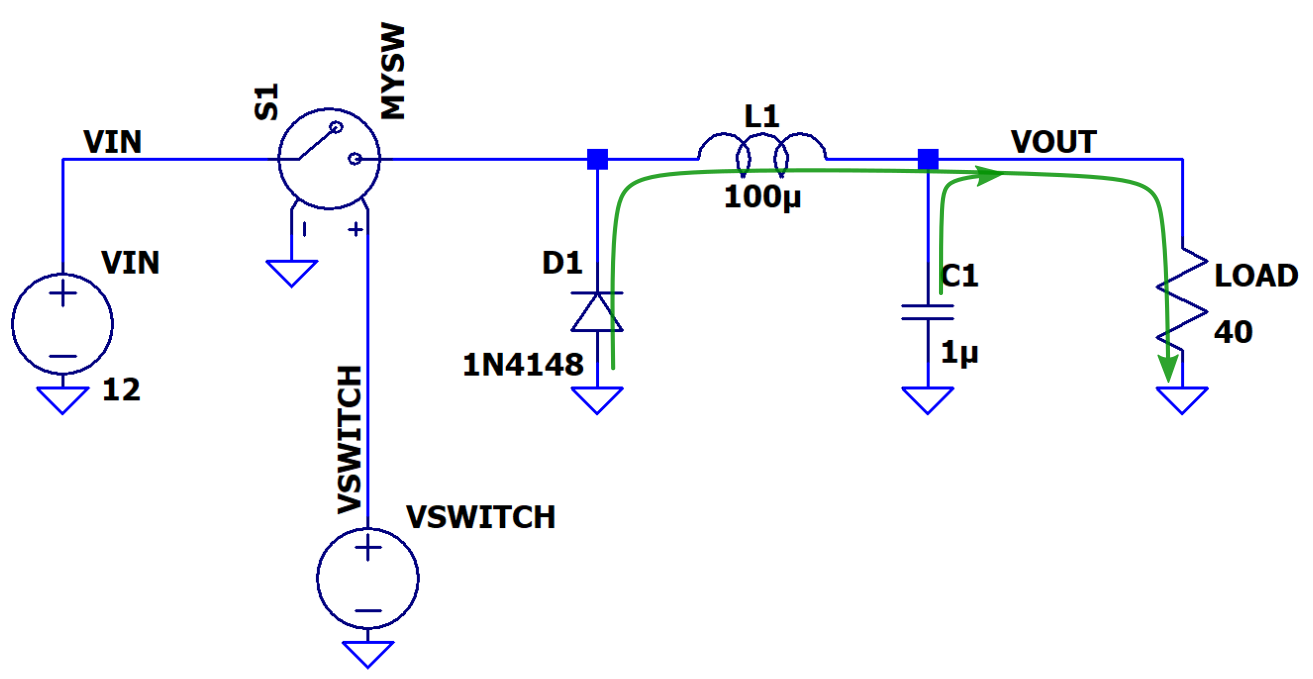##### Figure 4. Buck converter current flow in the Off state

When the switch turns off, the inductor, L1, functions like a source instead of a load. The inductor maintains current flow despite the loss of the input supply, but its current is ramping down.

When the inductor current decreases, the polarity of its voltage drop reverses relative to the polarity when the current is increasing. In the schematic of Figure 4, this means that the left-hand inductor terminal becomes negative relative to the right-hand terminal, and in fact, this voltage even becomes negative relative to ground.

When the left-hand inductor terminal voltage becomes negative enough to forward-bias the diode, D1, current can circulate as shown in the diagram. The diode plays a crucial role in a switch-mode regulator. It doesn’t interfere with circuit operation during the On state, and it creates a low-impedance path for inductor current during the Off state.

### Buck Converter Transient Response During Turn Off

Let’s look at the off-state version of the multi-pane plot shown in Figure 5, again working our way from the bottom to the top.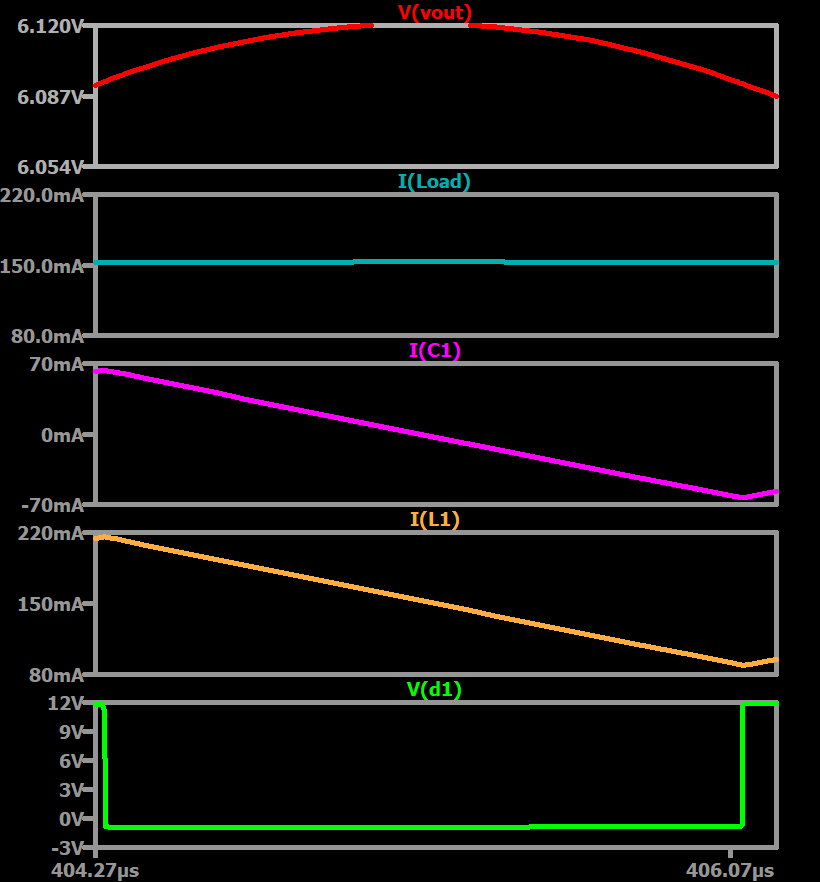#### Diode Voltage

Notice that V(d1), the voltage of the diode’s cathode and also the voltage of the left-hand terminal of the inductor, drops not to zero but below zero. It is far enough below zero to forward-bias the diode. The diode’s anode is tied directly to ground, and thus forward bias requires a negative voltage at the cathode.

#### Inductor Current

The inductor current, I(L1) is ramping down, indicating that the inductor is discharging, i.e., losing energy in its magnetic field. The inductor’s voltage drop is such that it can draw current from the ground node through the diode.

#### Capacitor Current

The capacitor current, I(C1), is decreasing and crosses from the positive region into the negative region at a moment corresponding to the transition from increasing capacitor voltage to decreasing capacitor voltage in the V(vout) plot.

As in the On state, the load current remains essentially constant during the Off state.

#### Output Voltage

The output voltage increases as the capacitor supplies charge to the load and then begins to decrease as the capacitor current becomes negative. Notice that the scale for the voltage is again exaggerated to highlight the relatively low output voltage variation.

### The Stable Output Voltage and Current of a Buck Converter

We’ve examined the electrical behavior of a step-down switching-regulator power stage during the switch-on state and the switch-off state. I hope that this discussion has helped you to understand how these circuits maintain stable voltage and consistent load current while also achieving high efficiency. This is made possible with the on/off switch control.

In the next article, we’ll consider component sizing and selection.